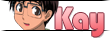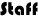View Single Post04-30-2011, 05:33 PM #1The Biggest Troll on the website   Join Date: Dec 2010 Location: South East Pennsylvania Posts: 434 Rep Power: 144Answers to the Math Problems! I said I post the answers for these math problems on the forums so here they are! Question One: This should be simple! 1. Add up all the genre totals (3+2+4+7+12+45= 73) 2. Multiply the total number of books times the average height to get the total height. (73 * .75 = 54.75) 3. Convert the height from inches to meters! 1 inches = 0.0254 meters FYI (54.75*.0254 = 1.39065 meters) Solution = 1.39065 meters Question Two: Looks hard but but is quite doable! 1.The question suddenly becomes a lot easier once you realize that if you have 10 hours, 1 hour out of that would be 10% or 1/10th. It make converting everything into time much easier. 2. Time to convert! Video Room (A quarter 1/4 = 2 hours, 30 minutes); Artist Alley (17.5% = 1 hour, 45 minutes); Panels (2 hours); Dealer's Room (1/20 = 30 minutes); Cosplayers (45 minutes); Bathroom (5%= 30 minutes) 3. Add that all together and you get 8 hours. Subtract that from 10 to get 2! Solution = 2 Hours Question Three: Super Simple! 1. Find out the total cost! Figure out the tax (60 * .06 = 3.60) and then add it to the original cost (60 + 3.60 = 63.60) 2. Find out the total number of minutes! Multiply the number of minutes times the number of episodes! (26 * 25 = 650) 3. Divide the minutes by the total cost! (63.6 / 650 = .09784) since we are dealing with money, round it up to 10 cents per minute! Solution = 10 cents per minute Question Four: Super Simple! 1. Figure out how many riceballs you need! Two riceballs for six people means (2 * 6 = 12) 2. Figure out how much rice you need! You're making 12 riceballs that are 125 grams each so (12 * 125 = 1500 grams) Solution 1500 grams Question Five: Trivia question! The exact answer of this question depends on how many episodes of Once Piece there is. As of April 30, 2011, there are 496 episodes out, but this number will keep growing for some time. The solutions will get bigger as time passes. The equations are (One Piece Episodes / 5) to get the number of weeks required if watching one episode every weekday (answer today, 99 weeks and a day, just a little less then 2 years) and (One Piece Episodes / 52) to figure out how many episodes to watch per week to watch them all in one year. That's 9 and a half episodes every week! Solutions: about 2 years watching every weekday / 9.5 episodes weekly to watch it all in one year Any questions?